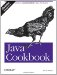# Recipe 13.4 Drawing Centered Text in a Component

#### Problem

You want to draw text neatly centered in a component.

#### Solution

Measure the width and height of the string in the given font, and subtract it from the width and height of the component. Divide by two, and use this as your drawing location.

#### Discussion

The program DrawStringDemo2 measures the width and height of a string (see Figure 13-2 for some attributes of the text). The program then subtracts the size of the text from the size of the component, divides this by two, and thereby centers the text in the given component.

`public class DrawStringDemo2 extends Component {     String message = "Hello Java";        /** Paint is called (by AWT) when it's time to draw the text. */      public void paint(Graphics g) {          // Get the current Font, and ask it for its FontMetrics.          FontMetrics fm = getFontMetrics(getFont( ));            // Use the FontMetrics to get the width of the String.          // Subtract this from width, divide by 2, that's our starting point.          int textX = (getSize( ).width - fm.stringWidth(message))/2;          if (textX<0)        // If string too long, start at 0              textX = 0;            // Same as above but for the height          int textY = (getSize( ).height + fm.getAscent( ))/2 - fm.getDescent( );          if (textY<0)              textY = 0;            // Now draw the text at the computed spot.          g.drawString(message, textX, textY);      }        public Dimension getPreferredSize( ) {          return new Dimension(100, 100);      }  }`

##### Figure 13-2. Font metricsThis is so common that you'd expect Java to have encapsulated the whole thing as a service, and in fact, Java does do this. What we have here is what most GUI component architectures call a label. As we'll see in Chapter 14, Java provides a Label component that allows for centered (or left- or right-aligned) text and supports the setting of fonts and colors. It also offers JLabel, which provides image icons in addition to or instead of text.

To draw formatted text as in a word processor requires considerably more complexity. You'll find an example in the online source under the JabberPoint program (see ShowView.java). This program also implements the Model-View-Controller pattern.Java Cookbook, Second Edition
ISBN: 0596007019
EAN: 2147483647
Year: 2003
Pages: 409
Authors: Ian F Darwin

Similar book on Amazon